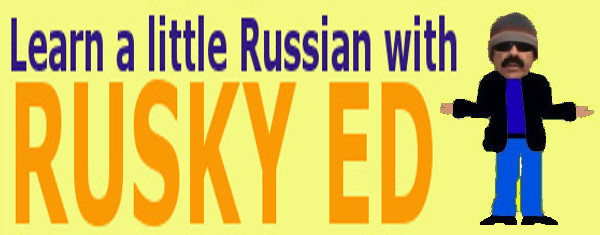Arithmetic - Miscellaneous
[ Number Sense ]    [ Addition and Subtraction ]    [ Multiplication and Division ]    [ Factors and Multiples ]    [ Decimal Operations ]    [ Fraction Operations ]    [ Miscellaneous ]    [ Home ]
 FEATURED VIDEOS:
```
```

## Arithmetic - Miscellaneous

Concentration - A memory game involving arithmetic facts!

Analog to Digital - Converting analog to digital time.

Time Stack - Adding a stack of time.

Pyramid Challenge - Single player strategy game.

Perimeter of Triangles - Calculating the perimeter of triangles.

Perimeter of Rectangles - Calculating the perimeter of rectangles.

Area of Rectangles - Calculating the area of rectangles.

Combined Rectangles One - Calculating the area of combined rectangles.

Combined Rectangles Two - Calculating the are of more complex shapes.

Trapeziums - Trapezium and trapezoid discussion and demo.[ Number Sense ]    [ Addition and Subtraction ]    [ Multiplication and Division ]    [ Factors and Multiples ]    [ Decimal Operations ]    [ Fraction Operations ]    [ Miscellaneous ]    [ Home ]